## Design a System in Simulink

Model-Based Design paradigm is centered on models of physical components and systems as a basis for design, testing, and implementation activities. This tutorial adds a designed component to an existing system model.

### Open System Model

The model is a flat robot that can move or rotate with the help of two wheels, similar to a home vacuuming robot. Open the model by entering in the MATLAB® command line:

```open_system('system_model.slx') ```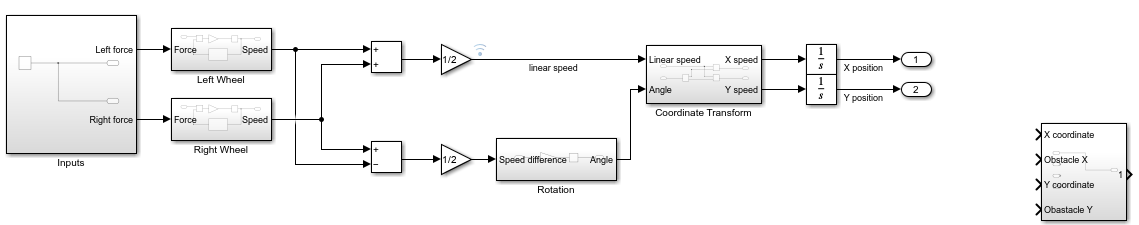This tutorial analyzes this system and adds functionality to it.

### Identify Designed Components and Design Goals

Specification of the design objective is a critical first step to the design task. Even with a simple system, there could be multiple and even competing design goals. Consider these goals for the example model:

• Design a controller that varies the force input so that the wheels turn at a desired speed.

• Design inputs that make the device move in a predetermined path.

• Design a sensor and controller so that the device follows a line.

• Design a planning algorithm so that the device reaches a certain point using the shortest path possible while avoiding obstacles.

• Design a sensor and algorithm so that the device moves over a certain area while avoiding obstacles.

This tutorial designs an alert system. You determine the parameters for a sensor that measures the distance from an obstacle. A perfect sensor measures the distance from an obstacle accurately. An alert system samples those measurements at fixed intervals so that the output is always within 0.05 m of the measurement. The system generates an alert in time for the robot to come to a stop before hitting the obstacle.

### Analyze System Behavior Using Simulation

The design of the new component requires analyzing the linear motion of the robot to determine:

• How far the robot can travel at the top speed when power to the wheels is cut

• The top speed of the robot

Simulate the model with a force input signal that starts motion, waits until the robot reaches a steady velocity, and then sets the force to zero:

1. In the model, double-click the subsystem named `Inputs`.

2. Delete the existing step input and add a Pulse Generator block.

3. Set these parameters for the Pulse Generator block:

• Amplitude: `1`

• Period: `20`

• Pulse Width: `15`

These parameters are designed to ensure that the top speed is reached. You can change parameters to see their effect.

4. Simulate the model for 20 seconds.

To analyze the simulation results, view the signals connected to the floating scopes in the model.

The first scope shows that the speed of the robot rapidly decreases after the pulse that represents the input force drops to zero at a simulation time of `3` seconds. The speed asymptotically approaches zero but never quite reaches zero. Accurate modeling for low speed dynamics without external forces requires a much more complex representation of the system. For the objective here, however, this approximate representation of the system is sufficient.The second scope shows the position of the robot over the course of the simulation. At the start, the position changes more rapidly. At about a simulation time of `3` seconds, the position changes more slowly as the speed of the robot decreases.Zoom in on the scope plot that shows the robot position. At time `3`, the position of the robot is approximately `0.55` m. At the end of the simulation, the position of the robot is approximately `0.7` m. Because the speed of the robot is very close to zero at the end of the simulation, the results show that the robot moves less than `0.16` m after the external force drops to zero.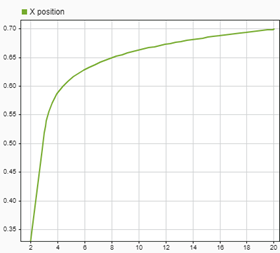To find the top speed:

1. Zoom in on the flat region of the velocity signal in time, from 1 s to 3 s.

2. Leave zoom mode by clicking the zoom button again.

3. Click Cursor Measurements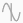.

4. Position the second cursor in the region where the velocity curve is flat.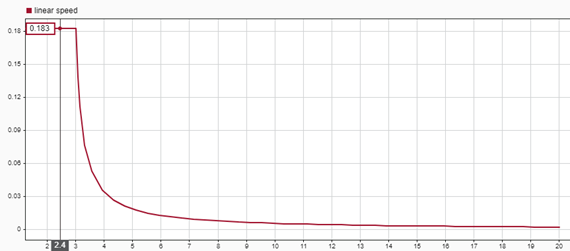The Value column in the Cursor Measurements panel indicates that the top speed of the robot is `0.183` m/s. To calculate the time it takes for the robot to travel 0.05 m, divide 0.05 m by 0.183 m/s to get the result of 0.27 sec.

### Design Components and Verify Design

Sensor design consists of these components:

• Measurement of the distance between the robot and the obstacle — This example assumes that the measurement is perfect.

• The time interval between each distance measurement the alert system makes — To keep the measurement error below 0.05 m, the sampling interval must be less than 0.27 sec. Use 0.25 sec.

• The distance at which the sensor produces an alert — Analysis shows that slow down must start by the time the robot is approximately 0.16 m from the obstacle. The actual alert distance must also take the error from discrete measurements, 0.05 m, into account.

Build the sensor:

1. Create a subsystem with four input ports and one output port. The subsystem receives inputs for the x- and y-coordinates of the robot and for the x- and y-coordinates of the obstacle. The alert signal produced by the sensor connects to the output port.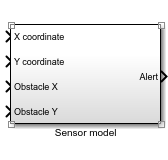2. Construct the distance measurement subsystem. In the subsystem named `Sensor model`, use the Subtract block, the Math Function block with the function `magnitude^2`, the Sum block, and the Sqrt block to implement the distance calculation. Notice that within the subsystem, the arrangement of the input ports does not need to match the arrangement of the ports on the Subsystem block interface.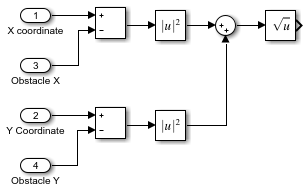3. To model the sampling, add a Zero-Order Hold block to the subsystem from the Discrete library and set the Sample time parameter for the block to `0.25`.

4. Connect the result of the distance calculation to the input of the Zero-Order Hold block.

5. To model the alert logic, add a Compare to Constant block from the Logic and Bit Operations library and set these block parameters:

• Operator: `<=`

• Constant Value: `0.21`

• Output data type: `boolean`

With these parameter values, the block output value is `1` when the input value is less than or equal to `0.21`.

6. Connect the output of the Zero-Order Hold block to the input of the Compare to Constant block.

7. Finally, connect the output of the Compare to Constant block to the Outport block named `Alert`.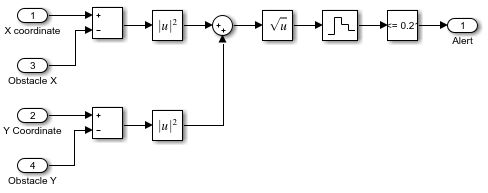#### Verify Design

Test the design with an obstacle location of X = 0.65, Y = 0 using Constant blocks as inputs to the Sensor model subsystem. This test verifies design functionality in the X direction. You can create similar tests for different paths. This model only generates an alert. It does not control the robot.

1. Set the obstacle location. Add two Constant blocks from the Sources library set the constant values to `0.65` and `0`. Connect the position outputs of the robot to the inputs of the sensor.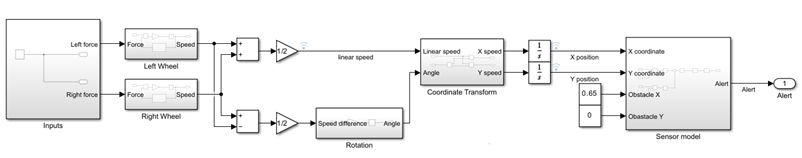3. Simulate the model.

The plot of the robot position in the scope looks the same as the previous run.The scope connected to the alert signal shows that the alert signal value becomes `1` when the robot comes within `0.21` m of the obstacle location, satisfying the design requirement for this component.For real-world systems with complex components and formal requirements, the Simulink® product family includes additional tools to refine and automate the design process. Requirements Toolbox™ provide tools to formally define requirements and link them to model components. Simulink Control Design™ can facilitate the design if you want to build a controller for this robot. Simulink Verification and Validation™ products establish a formal framework for testing components and systems.